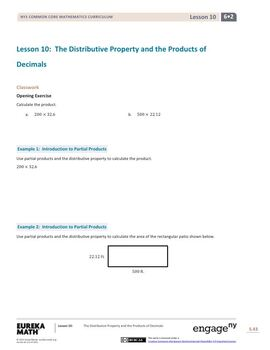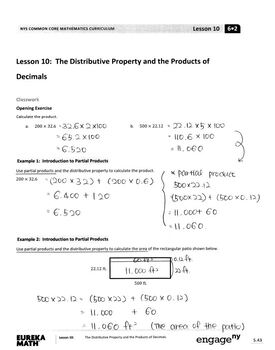# Engage NY - Eureka Math Grade 6 Module 2 Lesson 10 Student Workbook Answer KeysSubject
Resource Type
Product Rating
File Type

PDF

(18 MB|10 pages)
Product Description

Engage NY - Eureka Grade 6 Module 2 Lesson 10 Student Workbook Answer Keys with Solutions is strictly for HOMESCHOOLERS and PARENTS who want to help their children solve student workbook questions at home. This lesson includes Eureka Math student worksheets, and answer keys with detail explanation on how to solve. The answer keys are in black and white or in red, so that you can choose the version you find easy to read.

You also can purchase the bundle for the whole Grade 6 from Module 1 through Module 6 that contains all 6 modules and 142 lessons for grade 6 ENTIRE YEAR.

Work in progress....

Or you can find each module unit bundles as below

MODULE 1. Ratios and Unit Rates

(Lesson 1 through Lesson 29 Plus 29 Exit Tickets)

MODULE 2. Arithmetic Operations Including Division of Fractions

(Lesson 1 through Lesson 19 Plus 19 Exit Tickets)

MODULE 3. Rational Numbers

(Lesson 1 through Lesson 19 Plus 19 Exit Tickets)

MODULE 4. Expressions and Equations

(Lesson 1 through Lesson 34 Plus 34 Exit Tickets)

MODULE 5. Area, Surface Area, and Volume Problems

(Lesson 1 through Lesson 19 Plus 19 Exit Tickets)

MODULE 6. Statistics

(Lesson 1 through Lesson 22 Plus 22 Exit Tickets)

MODULE 2. Arithmetic Operations Including Division of Fractions

Topic A: Dividing Fractions by Fractions

Lesson 1: Interpreting Division of a Fraction by a Whole Number - Visual Models

Lesson 2: Interpreting Division of a Fraction by a Whole Number - Visual Models

Lesson 3: Interpreting and Computing Division of a Fraction by a Fraction—More Models

Lesson 4: Interpreting and Computing Division of a Fraction by a Fraction—More Models

Lesson 5: Creating Division Stories

Lesson 6: More Division Stories

Lesson 7: The Relationship Between Visual Fraction Models and Equations

Lesson 8: Dividing Fractions and Mixed Numbers

Topic B: Collections of Equivalent Ratios

Lesson 9: Sums and Differences of Decimals

Lesson 10: The Distributive Property and the Products of Decimals

Lesson 11: Fraction Multiplication and the Products of Decimals

Topic C: Unit Rates

Lesson 12: Estimating Digits in a Quotient

Lesson 13: Dividing Multi-Digit Numbers Using the Algorithm

Lesson 14: The Division Algorithm—Converting Decimal Division into Whole Number Division Using Fractions

Lesson 15: The Division Algorithm—Converting Decimal Division into Whole Number Division Using Mental Math

Topic D: Percent

Lesson 16: Even and Odd Numbers

Lesson 17: A Fraction as a Percent

Lesson 18: Percent of a Quantity

Lesson 19: Solving Percent Problems

Exit Tickets for Module 2

Eureka Math Grade 6 Module 2 Exit Tickets

(Lesson 1 through Lesson 19)

**After download and use, please rate this product. Your feedback is invaluable in the development of new products to better serve you. Thanks so much!**

Total Pages
10 pages
Included
Teaching Duration
2 hours
Report this Resource to TpT
Reported resources will be reviewed by our team. Report this resource to let us know if this resource violates TpT’s content guidelines.